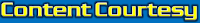# Sample Questions for CBSE Proficiency Test for Class 10thLatest Sample Questions for CBSE Proficiency Test for Class 10th
Download Latest Sample Papers for CBSE Proficiency Test Free!

1. SCIENCE :
• This document provides some general guidelines for the CBSE Proﬁciency Test in Science to be held in May-June, 2011. It aims to introduce the test to interested students and explains the format of the question paper.
• The CBSE Proﬁciency Test in Science aims to test higher level competencies in the subject which include:
– knowledge of the facts/laws/concepts/principles of the subject and appreciation of their
inter-relationship,
– ability to apply the above knowledge to understand relevant daily life situations,
– ability to apply the above knowledge to new and unfamiliar situations,
– ability to solve problems requiring the application of the above knowledge,
– ability to interpret numerical data, graphs, etc., and
– ability to understand experimental and measurement aspects of Science.
• The content level will be deﬁned by the prescribed CBSE class IX and class X syllabus. The recommended textbooks for this content are NCERT Science textbooks for classes IX and X.
• The questions in the test will not be based merely on recall of information given in the textbooks, and several of them may be unconventional.
• The maximum marks for the test will be 100 and the test will have a duration of two and half hours.
• The question paper will be fully objective in nature; answers will be machine-gradable. The paper will consist of multiple choice questions (MCQs), numerical questions and column matching questions as illustrated by the sample questions given below. There will be negative marking for wrong answers to the multiple choice questions only.
• The total number of questions is NOT indicated by the number of questions in the sample given here. The distribution of marks among different types of questions is NOT indicated by the number of questions of each type in the sample given here.

Click Below to Download (Science)

1. MATHEMATICS :

• This document provides some general guidelines for the CBSE Proﬁciency Test in Mathematics to be held in May-June, 2011. It aims to introduce the test to interested students and explains the format of the question paper.
• The CBSE Proﬁciency Test in Science aims to test higher level competencies in the subject which include:
– knowledge of concepts/principles and techniques of mathematics,
– ability to solve problems requiring the application and interconnection of the above knowledge, ability to apply the above knowledge to analyse relevant daily life situations and draw appropriate inferences,
– ability to solve problems requiring numerical data-interpretation, graphical interpretation, etc., and
– ability to reason mathematically.
• The content level will be deﬁned by the prescribed CBSE class IX and class X syllabus. The recommended textbooks for this content are NCERT Mathematics textbooks for classes IX and X.
• The questions in the test will not be based merely on recall of information given in the textbooks, and several of them may be unconventional.
• The maximum marks for the test will be 100 and the test will have a duration of two and half hours.
• The question paper will be fully objective in nature; answers will be machine-gradable. The paper will consist of multiple choice questions (MCQs), numerical questions and column matching questions as illustrated by the sample questions given below. There will be negative marking for wrong answers to the multiple choice questions only.
• The total number of questions is NOT indicated by the number of questions in the sample given here. The distribution of marks among different types of questions is NOT indicated by the number of questions of each type in the sample given here.

Click Below to Download (Mathematics)cbse.nic.in
Disclaimer: There should be a rigid understanding among the readers that the content available here is only for the purpose of providing academic help to our esteemed readers (students) and the same is originally available on cbse.nic.in.

### 1 comment

1.thank u sir

#### Post a Comment

Subscribe Our Newsletter